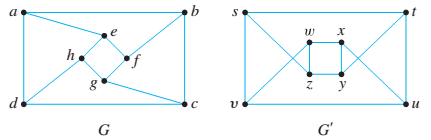Chapter 10.3, Problem 12ES### Discrete Mathematics With Applicat...

5th Edition
EPP + 1 other
ISBN: 9781337694193

#### Solutions

Chapter
Section### Discrete Mathematics With Applicat...

5th Edition
EPP + 1 other
ISBN: 9781337694193
Textbook Problem
1 views

# For each pair of simple graphs G and G ′ in 6—13, determine whether G and G ′ are isomorphic. If they are, give a function g : V ( G ) → V ( G ′ ) that defines the isomorphism. If they are not, give an invariant for graph isomorphism that they do not share. 12.To determine

Whether the given two graphs are isomorphic to each other or not. If not give the invariant of them.

Explanation

Given information:

The given two graphs G and G' are as follows-

Calculation:

The two graphs G and G' are isomorphic if and only if there exists one-to-one correspondence between the vertices and edges of the graphs. Mathematically, if V(G) be the vertex set, E(G) be the edge set of G and V(G'), E(G') be the vertex set and edge set of G', and there exi

### Still sussing out bartleby?

Check out a sample textbook solution.

See a sample solution

#### The Solution to Your Study Problems

Bartleby provides explanations to thousands of textbook problems written by our experts, many with advanced degrees!

Get Started